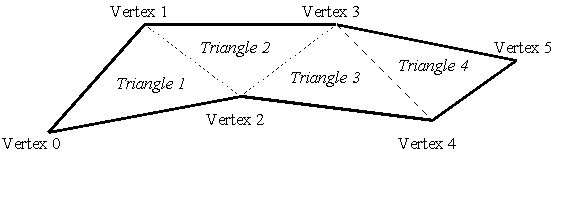Draw Gouraud Polytriangle

Purpose

The Draw Gouraud Polytriangle function draws a connected chain of smoothly (Gouraud) shaded triangles.

Syntax

 Opcode format Opcode [ASCII] (Hex) Operand Format Comments Extended ASCII (Gouraud ,,,,,,,,[,,,,]+[]) Absolute coordinates. Single-byte, binary operand [g] (67) [][]+ Relative coordinates. [Ctrl-G] (07) [][]+ Relative coordinates.

Count The number of triangles to be drawn, which is two less than the number of vertices included. In the binary operand case, a value of zero indicates that an extended count will follow. In the extended ASCII case, count may be any value larger than zero.

Ecount When count is zero, a two-byte extended count follows. This allows for Polytriangles of length 256 through 65,791 which are encoded as an integer in the range 0 to 65,535.

X1,Y1 The first vertex (in logical coordinates) of the first triangle in the list.

R1,G1,B1,A1 The color of the first vertex of the first triangle in the list (interpreted as intensities of the red, green, blue, and alpha channels, respectively). Legal intensity values range from zero to 255.

C1 The color of the first vertex of the first triangle in the list (interpreted as 4 bytes representing an RGBA color).

X2,Y2 The second vertex (in logical coordinates) of the first triangle in the list.

R2,G2,B2,A2 The color of the second vertex of the first triangle in the list.

C2 The color of the second vertex in the list (interpreted as four bytes representing an RGBA color).

Xi,Yi A vertex (in logical coordinates) in the list that, when combined with the previous two vertices, defines the ith triangle in the list.

Ri,Gi,Bi,Ai The color of the second vertex of the first triangle in the list.

Ci The color of the ith vertex in the list (interpreted as 4 bytes representing an RGBA color).

Details

Topologically, a polytriangle is a strip of connected triangles, where each successive triangle in the chain is defined by a single vertex and two vertices from the previous triangle in the strip as shown in figure 1.Figure 1. polytriangle of six points

A polytriangle is similar to a polygon, but the polytriangle vertices are specified in a special order that allows for well-defined smooth shading of the triangle interiors.

When the "Fill Mode" attribute is inactive (the default) the edges of the triangles are rendered as Gouraud shaded lines in accordance with the current "Line Weight", "Line Cap", "Line Pattern", and "Visibility" attributes.

When the "Fill Mode" attribute is active, each triangle is rendered by smoothly interpolating the color from each vertex across the interior.

Notes

If a Gouraud Polytriangle with more than 65,791 triangles is desired, it must be drawn by multiple Draw Gouraud Polytriangle opcodes.

Implementation in WHIP! Versions

 Toolkit Function TBD Partial Full Revised in Draw Gouraud Polytriangle - - - -